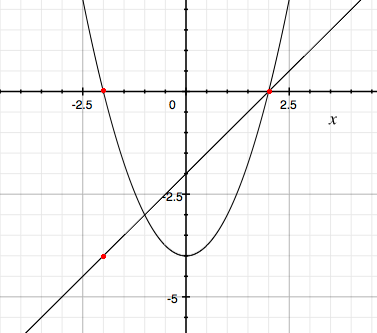SEARCH HOMEMath Central Quandaries & QueriesQuestion from sarah, a student: f(x)={x-2, x<-2        {x^2-4, -2_2 find the rangeHi Sarah,

I would plot the three functions

\begin{eqnarray*}
p(x) & = & x-2 \\
q(x) &=&x^2 - 4\\
r(x) & =& x + 2.
\end{eqnarray*}

on the same piece of paper and then erase the parts of the graphs that fall outside the appropriate ranges. I will plot $p(x)$ and $q(x).$I put a red dot on $p(x) = x-2$ at $(-2, -4)$ since $f(x)$ is only equal to $p(x)$ for $x < -2.$ Erase the portion of the line $y = p(x)$ to the right of the red dot.

I put dots on the parabola $q(x) = x^2 -4$ at $x = -2$ and $x = 2$ since these points mark the ends of the interval where $f(x)$ coincides with $q(x).$ Erase the portions of the parabola that are outside the domain $-2 \leq x \leq 2.$

Now add the third function $r(x) = x+2$ and erase the part not in the domain of $f(x).$

Can you tell from the resulting graph the range of $f(x)?$

PennyMath Central is supported by the University of Regina and the Imperial Oil Foundation.